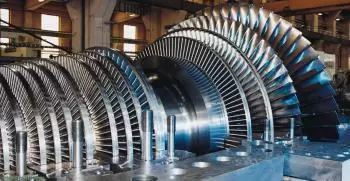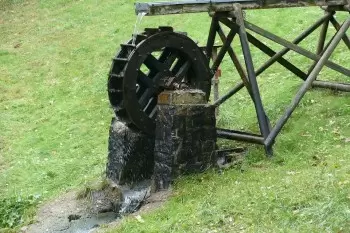# What Is Mechanical Energy?

Mechanical energy can be defined as the ability to produce mechanical work that a body possesses due to causes of mechanical origin, such as its position or its speed. There are two forms of mechanical energy that are:• kinetic energy
• potential energy

The mechanical energy of a body is the sum of its kinetic energy and its potential energy.

This type of energy can be partially transformed into other types of energy, such as electrical energy (in a fan), and can be obtained by transforming other energies, such as chemical energy (in a person who walks).

The kinetic energy of a body is associated with its velocities, linear and angular, and its mass). Actually, kinetic energy is the energy you are using at any given time. Potential energy, as the word itself indicates, is the energy you can potentially get.

### Examples of mechanical energy

For example, if we hold a ball in our hands it has the potential energy equivalent to the kinetic energy that it would have if we released it. If we release the ball and it falls, it will begin to acquire speed and therefore kinetic energy. As kinetic energy increases, potential energy decreases. In this case, the mechanical energy is always kept constant.

Today, many technological devices and machines convert mechanical energy into other forms of energy or vice versa. Of these devices we highlight the following categories:

• An electric motor converts electrical energy into mechanical energy. By supplying electric current to the motor we obtain a mechanical movement, the rotation of an axis. This axis, being an element with a certain mass and speed (angular velocity) has kinetic energy
• A generator converts mechanical energy into electrical energy. It is the same case of the electric motor, but in the opposite direction.
•A hydroelectric power station converts the mechanical energy of the water in a storage dam into electrical energy. Before opening the gates, all the water accumulated behind the gate has potential energy. When you open the gate, the water comes out under pressure with a certain speed, therefore, it is converted into kinetic energy. The outgoing water interacts with a turbine that will be in charge of converting kinetic energy into electrical energy. It is what we call hydraulic energy.
• An internal combustion engine is a thermal engine that draws mechanical energy from chemical energy by burning fuel (diesel, gasoline, etc.). In the case of a car, this mechanical energy is used to move. In the case of a thermal power plant, it is used.
• A steam engine converts the thermal energy of the steam into mechanical energy. In this case you can also analyze how the steam is obtained. To convert water into steam, heat energy is needed, this energy can be obtained in many ways, by thermal solar energy (unusual in these cases) or by burning fossil fuels such as coal, for example.
• A turbine converts the kinetic energy of a gas or liquid stream into mechanical energy. As we have commented in the case of hydraulic energy.

## Distinction of other types of energy with mechanical energy

The classification of energy into different types often follows the limits of the fields of study in the natural sciences.

Chemical energy is the type of potential energy stored in chemical bonds and is studied in chemistry. An example is gunpowder. By supplying a little heat to the powder, an exothermic chemical reaction occurs, that is, with the release of energy to the outside.

Nuclear energy is energy stored in the interactions between the particles in the atomic nucleus and is studied in nuclear physics.

Electromagnetic energy is in the form of electric charges, magnetic fields, and photons. It is studied in electromagnetism.

Various forms of energy in quantum mechanics; for example, the energy levels of the electrons in an atom.

Author:

Published: November 30, 2016
Last review: August 22, 2018